Next: Hyperfine Structure Up: Time-Independent Perturbation Theory Previous: Fine Structure of Hydrogen

# Zeeman Effect

Consider a hydrogen atom placed in a uniform-directed external magnetic field of strength. The modification to the Hamiltonian of the system is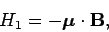(992)

where(993)

is the total electron magnetic moment, including both orbital and spin contributions [see Eqs. (758)-(760)]. Thus,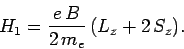(994)

Suppose that the applied magnetic field is much weaker than the atom's internal magnetic field (977). Since the magnitude of the internal field is about 25 tesla, this is a fairly reasonable assumption. In this situation, we can treat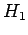as a small perturbation acting on the simultaneous eigenstates of the unperturbed Hamiltonian and the fine structure Hamiltonian. Of course, these states are the simultaneous eigenstates of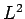,,, and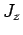(see previous section). Hence, from standard perturbation theory, the first-order energy-shift induced by a weak external magnetic field is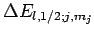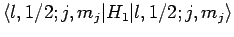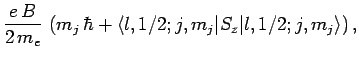(995)

since. Now, according to Eqs. (825) and (826),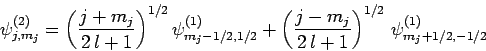(996)

when, and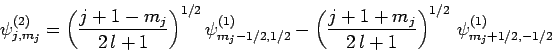(997)

when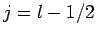. Here, the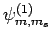are the simultaneous eigenstates of,,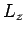, and, whereas theare the simultaneous eigenstates of,,, and. In particular,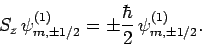(998)

It follows from Eqs. (996)-(998), and the orthormality of the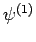, that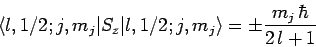(999)

when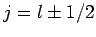. Thus, the induced energy-shift when a hydrogen atom is placed in an external magnetic field--which is known as the Zeeman effect--becomes(1000)

where the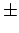signs correspond to. Here,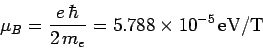(1001)

is known as the Bohr magnetron. Of course, the quantum number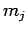takes values differing by unity in the rangeto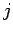. It, thus, follows from Eq. (1000) that the Zeeman effect splits degenerate states characterized byintoequally spaced states of interstate spacing(1002)

Likewise, the Zeeman effect splits degenerate states characterized byintoequally spaced states of interstate spacing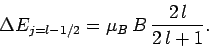(1003)

In conclusion, in the presence of a weak external magnetic field, the two degeneratestates of the hydrogen atom are split by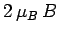. Likewise, the four degenerate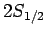andstates are split by, whereas the four degenerate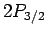states are split by. This is illustrated in Fig. 24. Note, finally, that since the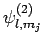are not simultaneous eigenstates of the unperturbed and perturbing Hamiltonians, Eqs. (1002) and (1003) can only be regarded as the expectation values of the magnetic-field induced energy-shifts. However, as long as the external magnetic field is much weaker than the internal magnetic field, these expectation values are almost identical to the actual measured values of the energy-shifts.Next: Hyperfine Structure Up: Time-Independent Perturbation Theory Previous: Fine Structure of Hydrogen
Richard Fitzpatrick 2010-07-20